# Carbon tetrabromide (CBr4) lewis dot structure, molecular geometry, polarity, hybridization, bond angle

Home  > Chemistry Article > CBr4 lewis structure and its molecular geometryCarbon tetrabromide also knows as tetrabromomethane is a colorless crystalline solid having the chemical formula CBr4. It consists of one carbon followed by four bromine atoms. It has a sweet odor and is soluble in ether, chloroform, ethanol, etc.

In this tutorial, we will discuss carbon tetrabromide(CBr4) lewis structure, molecular geometry, polar or nonpolar, hybridization, bond angle, etc.

Carbon tetrabromide is toxic by ingestion and uses to make other chemicals. It is used as a solvent for greases, waxes, and oils. It has a very high density, so, it is used also for separating minerals from various impurities.

Properties of Carbon tetrabromide

• It has a molar mass of 331.627 g·mol−1.
• It is insoluble in water.
• It has a boiling point of 189.7 °C and a melting point of 94.5 °C.
• It is denser than water.
• It has a monoclinic tetrahedral structure.
 Name of Molecule Carbon tetrabromide Chemical formula CBr4 Molecular geometry of CBr4 Tetrahedral Electron geometry of CBr4 Tetrahedral Hybridization Sp³ Nature Nonpolar Total Valence electron for CBr4 32
Page Contents

## How to draw lewis structure for CBr4?

CBr4 lewis structure contains carbon atom at middle position whereas four bromine atoms surrounding to it. A total of 12 lone pairs and 4 bonded pairs are present in the lewis structure of CBr4.

The lewis structure of CBr4 is similar to CCl4 and CF4, since, they all are in the same group in the periodic table and contains the same number of valence electrons.

## Follow some steps for drawing the lewis dot structure of CBr4

1. Count total valence electron in CBr4

Finding the total number of valence electrons in the CBr4 molecule is the first step for drawing its lewis diagram. “A valence electron is the outermost shell electrons around an atom”. To get the valence electron of an atom, look at its periodic group.

Since we have to find the valence electron in the CBr4 molecule, so, look at the periodic group of carbon and bromine atoms.

Carbon atoms belong to the 14th periodic group whereas bromine atoms are situated in the 17th group, hence, the valence electron for the carbon atom is 4, and for bromine, it is 7.

⇒ Total number of the valence electrons in carbon = 4

⇒ Total number of the valence electrons in bromine = 7

∴ Total number of valence electrons available for drawing the lewis structure of CBr4 = 4 + 7(4) = 32 valence electrons         [∴CBr4 molecule has one carbon and four bromine atom]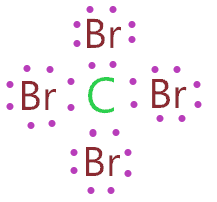2. Find the least electronegative atom and placed it at center

As we know, electronegativity increase as we move from left to right in the periodic table. Hence, the bromine atom is more electronegative than a carbon atom. Therefore, it can never be placed in a central position because it is less prone to share electrons.

So, place the carbon in the middle position and spread the four bromine atom around it.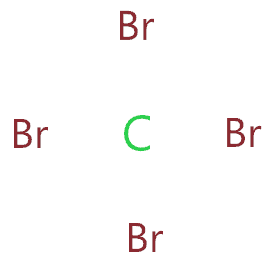3. Connect outer atoms to central atom with a single bond

Now join each bromine atom to the carbon central atom using a single bond.Now look at the above structure and count the number of valence electrons we used till now. A single bond means 2 shared pair electrons. In the above structure, four single bonds are used for connecting each bromine atom to a carbon central atom.

Hence, (4 × 2) = 8 valence electrons are used from a total of 32 valence electrons that are available for drawing the lewis structure of CBr4.

∴ (32 – 8) = 24 valence electrons

So, we are left with 24 valence electrons more.

4. Place remaining valence electrons starting from outer atom first

In this step, we have to put the remaining valence electron over each outer atom to complete their octet first. We know, in the CBr4 molecule, bromine is the outer atom, hence, each bromine atom needs 8 electrons in its outer shell to complete the octet and attains stability.

So, just start putting out the remaining valence electron around each bromine atom until they complete their octet.So, by looking at the above structure, we see each bromine atom gets 6 valence electrons represented as dots, and they also have 2 valence electrons in form of a single bond that is attached to them.

Hence, (6 valence electrons as dots + one single bond that contains 2 electrons) = 8 valence electrons are present around each bromine atom, hence, they all completed their octet.

5. Complete central atom octet and make covalent bond if necessary

This step is not required at all for making the lewis structure of CBr4 because the carbon central atom already completed its octet in the 4th step structure as 4 single bonds(that contains 8 electrons) are attached to the carbon atom.

Hence, each atom (carbon and bromine) completed its octet by having 8 valence electrons around them and we also used all 32 valence electrons that are available for drawing the lewis structure of CBr4.### CBr4 lewis structure

Now we will check the stability of the CBr4 lewis structure by using the formal charge concept.

6. Check the stability with the help of a formal charge concept

“The lesser the formal charge on atoms, the better is the stability of the lewis diagram.”

To calculate the formal charge on an atom. Use the formula given below-

⇒ Formal charge = (valence electrons – lone pair electrons –  1/2 bonded pair electrons)

Let’s start with the central atom which is carbon in the CBr4 molecule.

For carbon atom:

⇒ Valence electrons of carbon = 4

⇒ Lone pair electrons on carbon = 0

⇒ Shared pair electrons around carbon(4 single bond) = 8

∴ (4 – 0 – 8/2) = 0 formal charge on the carbon central atom.

Each bromine atom in the CBr4 lewis structure has the same number of lone pair and bonded pair electrons, hence, just count formal charge for just one bromine atom

For bromine atom

⇒ Valence electrons of bromine = 7

⇒ Lone pair electrons on bromine = 6

⇒ Shared pair electrons around bromine (1 single bond) = 2

∴ (7 – 6 – 2/2) = o formal charge on each bromine atom.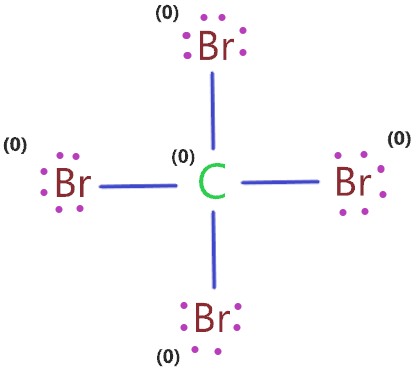So, each atom gets the formal charge equal to zero, hence, the above lewis structure of CBr4 is most stable and reliable.

## What is the molecular geometry of CBr4?

The molecular geometry of CBr4 is tetrahedral as the carbon central atom has no lone pair and is attached to the four bromine atoms with the help of a single bond. So, there are four regions of electron density around the carbon central atom”.

The electron pair around the carbon central atom will repel each other and tried to go far from each other, they will take the position where repulsion becomes minimum between them.

According to the VSEPR theory, “in most of the cases, the central atom with four regions of density adopt a tetrahedral structure because repulsion is minimum in electron pairs at this position.”

Hence, the final molecular geometry of CBr4 appears like a regular tetrahedron with a bond angle ∠BrC−Br =109.5°.

The carbon atom is located in the center of the tetrahedron, while the four bromine atoms are located on the vertices.### CBr4 molecular geometry

We can also find the electron and molecular geometry of CBr4 using the AXN method and VSEPR chart.

AXN is a simple formula that represents the number of the bonded atom and lone pair on the central atom to predict the shape of the molecule using the VSEPR chart.

AXN notation for CBr4 molecule:

• A denotes the central atom, so, carbon is the central atom in CBr4 molecule A = Carbon
• X denotes the bonded atoms to the central atom, carbon is bonded with four bromine atoms. Therefore, X = 4
• N represents the lone pair on the central atom, as per CBr4 lewis structure, the carbon central atom has no lone pair. Hence, N = 0

So, the AXN generic formula for the CBr4 molecule becomes AX4N0 or AX4.

As per the VSEPR chart, if a molecule gets AX4 generic formula then its molecular geometry will be tetrahedral and electron geometry will also be tetrahedral.

Look at the VSEPR chart below to clear your doubts.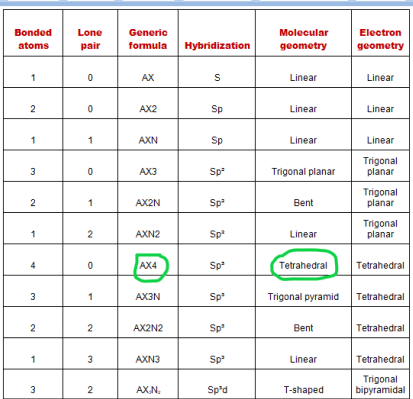## What is the Hybridization of CBr4?

“Hybridization is a theory that helps us understand the shape of molecular orbitals upon bonding to for compounds”

We will not go for the deep touch of the hybridization concept, we just want to know how to find the hybridization of the CBr4 molecule.

Just catch the steric number of the CBr4 molecule to gets it hybridized.

Steric number is the addition of a total number of bonded atoms around a central atom and the lone pair present on it.”

∴ Steric number of CBr4 = (Number of bonded atoms attached to carbon + Lone pair on carbon atom)

According to the lewis structure of CBr4, the carbon central atom is bonded with four bromine atoms and it contains no lone pairs.

∴ Steric number of CBr4 = (4 + 0) = 4

 Steric number Hybridization 1 S 2 Sp 3 Sp² 4 Sp³ 5 Sp³d 6 Sp³d²

So, we get the Sp3 hybridization for the CBr4 molecule because the steric number for the carbon central atom is 4.

## What is the bond angle of CBr4?

As we know the molecular geometry of CBr4 is tetrahedral, hence, according to the VSEPR theory, for a tetrahedral structure, the bonded atoms around the central atom will spread at an angle of approx 109.5° to minimize the repulsion and attains stability.

Hence, the bond angle of CBr4 is approx 109.5º.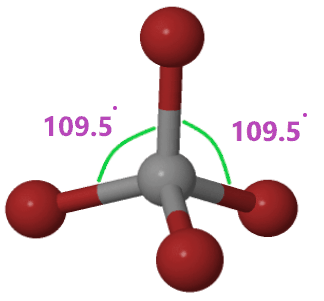## Carbon tetrabromide polarity: is CBr4 polar or nonpolar?

A polar molecule has an unequal distribution of charges on atoms which results in some net dipole moment, a polar bond generally occurs when the difference of electronegativity between the atoms is lies between 0.4 to 1.4 according to the Pauling scale.

A nonpolar molecule has an equal distribution of charges which results in a zero dipole moment because each dipole generated along the bond will cancel out each other, a nonpolar bond occurs when the difference of electronegativity between atoms is less than 0.4 according to the Pauling scale.

So, Is CBr4 polar or nonpolar? CBr4 is a nonpolar molecule although its bond(C-Br) is polar in nature because the difference in electronegativity between value bromine(2.96) and carbon(2.55) is 0.41 which lies in the range of the Pauling scale of electronegativity for the polar bonds.

It doesn’t mean if the bonds of any molecule are polar then the molecule will also be polar, the polar or nonpolar depends upon the overall dipole moment which is a case of CBr4 molecule is zero because of its symmetrical structure that causes the dipole generated along the bond(C-Br) canceled out each other.

CBr4 is in a tetrahedral molecular shape, so all the dipoles of the 4 polar C-Br bonds cancel each other out, resulting in an overall nonpolar molecule.

## FAQ

### Why the bond of CBr4 is polar but the whole molecule is nonpolar in nature?

The bonds(C-Br) are polar because the bromine atom is higher electronegative than the carbon atom, hence, it gets a negative charge whereas the carbon atom gets a slightly positive charge.

Due to the difference in electronegative value between bromine and carbon, the polarity is generated in the C-Br bond.

But the molecular geometry of CBr4 is tetrahedral which is symmetrical and all dipoles of C-Br bonds are opposite in direction to each other.

Hence, due to the symmetry of CBr4, all dipole gets canceled by each other leaving this molecule with net dipole moment equal to zero which makes it nonpolar in nature.

### What are the total lone pair and bond pairs present in the CBr4 lewis structure?

There is no lone pair on the carbon central atom but each outer atom(bromine) has 3 lone pairs on it. Hence, (4 bromine atoms × 3) = a total of 12 lone pairs.

And there are a total of 4 bonded pairs(single bonds) present in the CBr4 lewis structure

## Summary

• The total valence electron is available for drawing the carbon tetrabromide (CBr4) lewis structure is 32.
• The hybridization of CBr4 is Sp3 and the bond angle of 109.5°.
• CBr4 is a nonpolar molecule because of the zero net dipole moment caused by its symmetrical structure.
• In the CBr4 lewis structure, a total of 12 unshared and 4 shared pairs are present.
• The electron and molecular geometry of CBr4 are tetrahedral as per VSEPR theory.
##### Subscribe to Blog via Email

Join 2 other subscribers

Share it...